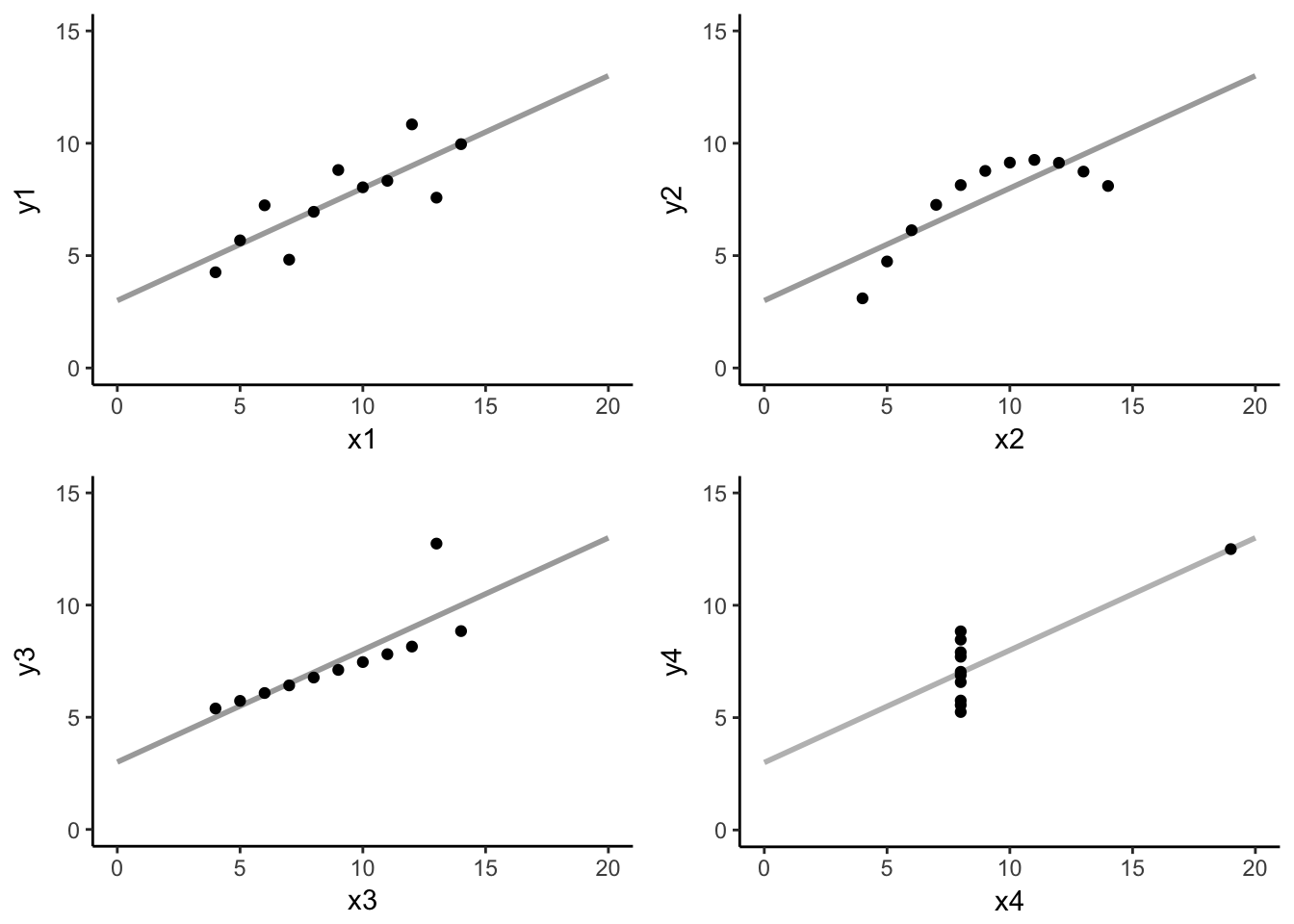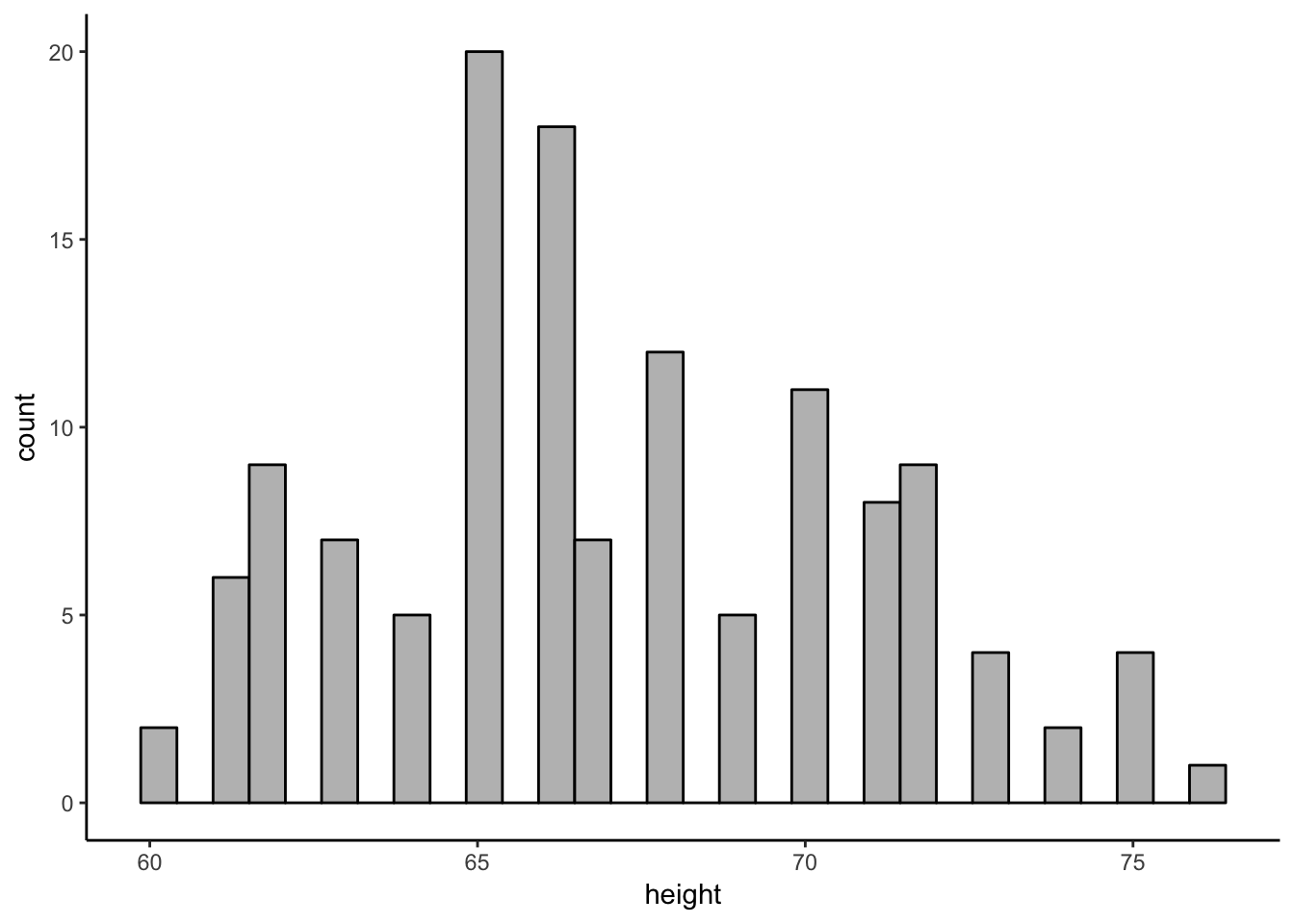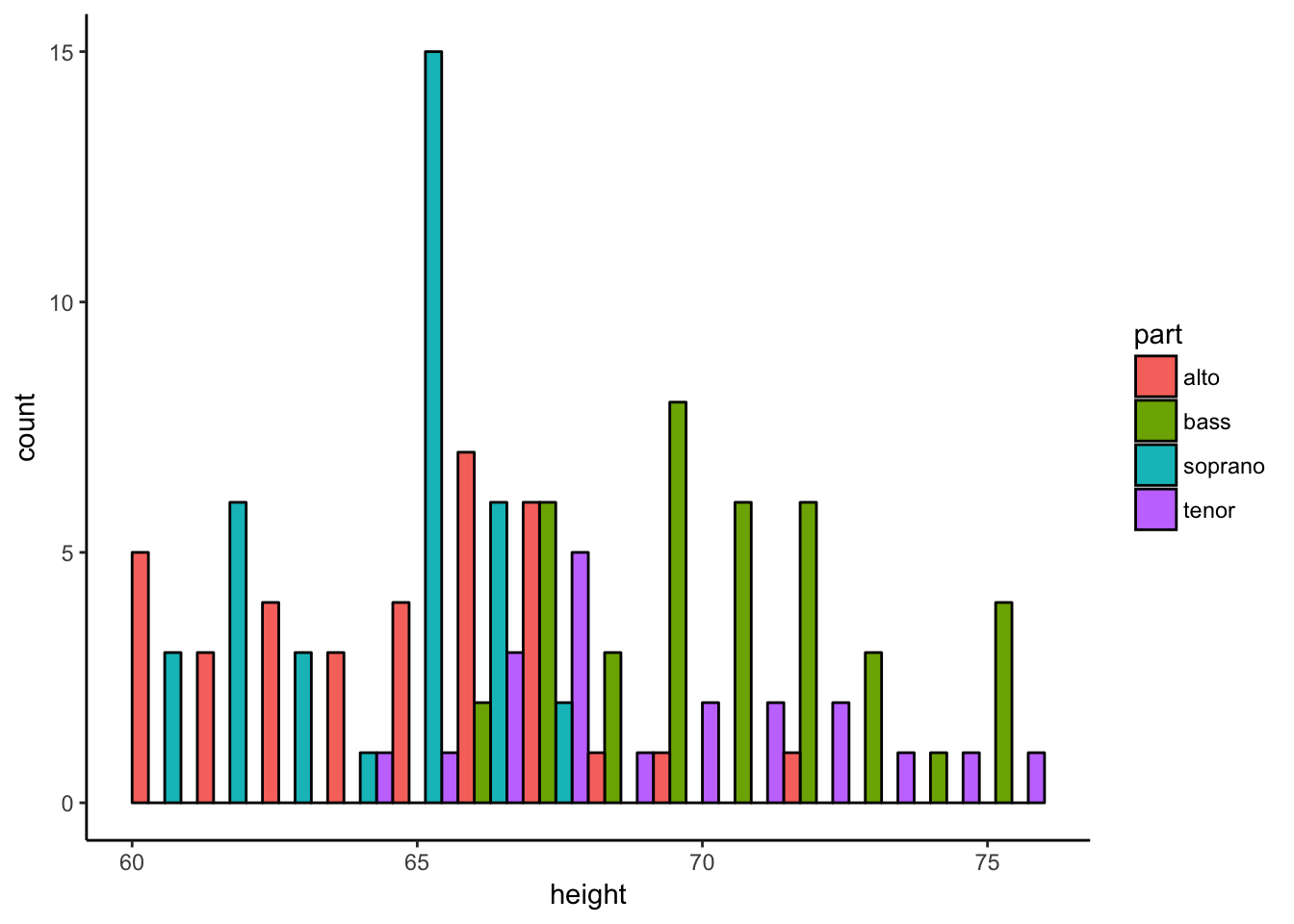## Preamble

The purpose of today’s section is to discuss one of the more powerful tools in our data analysis toolkit: visualization. You will learn:

• Why it is always a good idea to visualize your data
• The principles of creating effective and truthful data visualizations
• How to interpret some of the more common data visualizations
• How to identify misleading statistical graphics
``````# uncomment the next line to install the packages
# install.packages(c("cowplot", "tidyr", "ggthemes"))
library(dplyr)
library(tidyr)
library(stringr)
library(ggplot2)
theme_set(theme_classic())``````

## Why visualize data?

Data can be complex! But when we show data using graphical properties, such as size, shape or color, we are engaging in a form of visual communication that can help us (and others) understand large amounts of information very efficiently. Moreover, a good visualization can reveal information about the data that would be difficult to perceive otherwise.

To illustrate why visualization should almost always be the first step in an effective data analysis, let’s take a look at a dataset created by Francis Anscombe in 1973 for an article he described as trying to attack the impression among statisticians that “numerical calculations are exact, but graphs are rough.”

### A pathological example: Anscombe’s quartet

First, let’s take a look at the structure of the dataset using the `str()` function.

``str(anscombe)``
``````## 'data.frame':    11 obs. of  8 variables:
##  \$ x1: num  10 8 13 9 11 14 6 4 12 7 ...
##  \$ x2: num  10 8 13 9 11 14 6 4 12 7 ...
##  \$ x3: num  10 8 13 9 11 14 6 4 12 7 ...
##  \$ x4: num  8 8 8 8 8 8 8 19 8 8 ...
##  \$ y1: num  8.04 6.95 7.58 8.81 8.33 ...
##  \$ y2: num  9.14 8.14 8.74 8.77 9.26 8.1 6.13 3.1 9.13 7.26 ...
##  \$ y3: num  7.46 6.77 12.74 7.11 7.81 ...
##  \$ y4: num  6.58 5.76 7.71 8.84 8.47 7.04 5.25 12.5 5.56 7.91 ...``````

The data consist of 8 sets of 11 numeric observations (in x,y pairs) named X1, X2, X3, X4 and Y1, Y2, Y3, Y4.

Next, we are going to do some minor data wrangling to make it easier to compute summary statistics using our `dplyr` skills.

``````# no need to worry about the details of what this code is doing
# but basically we are extracting the variable type (x vs. y) and the number (1-4)
# and separting them into two columns
ansc_df <- anscombe %>%
gather(key = name, value = value) %>%
mutate(number = str_extract(name, pattern = "[:digit:]"),
variable = str_extract(name, pattern = "[:alpha:]")) ``````

Ok, now that we have cleaned up our data, let’s start with something reasonable and “summarize” the Anscombe dataset by calculating (a) the number of observations, (b) a measure of central tendency (the mean), and (c) a measure of variability (the standard deviation) for all 8 X and Y variables.

``````# use the summarise() function to compute the mean and standard deviation
# for each set of observations
summaries <- ansc_df %>%
group_by(variable, number, name) %>%
summarise(n_obs = n(),     # n observations
m = mean(value), # central tendency
sd = sd(value))  # variability
# print the summary table
summaries %>%
ungroup() %>%
select(name:sd)``````
``````## # A tibble: 8 x 4
##    name n_obs        m       sd
##   <chr> <int>    <dbl>    <dbl>
## 1    x1    11 9.000000 3.316625
## 2    x2    11 9.000000 3.316625
## 3    x3    11 9.000000 3.316625
## 4    x4    11 9.000000 3.316625
## 5    y1    11 7.500909 2.031568
## 6    y2    11 7.500909 2.031657
## 7    y3    11 7.500000 2.030424
## 8    y4    11 7.500909 2.030579``````

Discussion: What do we see in this table? Do we think these sets of X/Y observations are different from each other?

But, before we go tell anybody about our finding, let’s visualize the data.

``````a <- anscombe %>%
ggplot(., aes(x = x1, y = y1)) +
geom_smooth(se = F, method = "lm", fullrange = T, color = "darkgrey") +
geom_point() +
xlim(0, 20) +
ylim(0,15)

b <- anscombe %>%
ggplot(., aes(x = x2, y = y2)) +
geom_smooth(se = F, method = "lm", fullrange = T, color = "darkgrey") +
geom_point() +
xlim(0, 20) +
ylim(0,15)

c <- anscombe %>%
ggplot(., aes(x = x3, y = y3)) +
geom_smooth(se = F, method = "lm", fullrange = T, color = "darkgrey") +
geom_point() +
xlim(0, 20) +
ylim(0,15)

d <- anscombe %>%
ggplot(., aes(x = x4, y = y4)) +
geom_smooth(se = F, method = "lm", fullrange = T, color = "grey") +
geom_point() +
xlim(0, 20) +
ylim(0,15)

cowplot::plot_grid(a, b, c, d)``````Whoa! What’s going on here? All four datasets are identical when examined using simple summary statistics, but vary considerably when plotted!

[Note: the position along the x axis is different than the plots we’ve been looking at in class for SS, where the points were spread out along the x-axis to show some separation between points but this x position didn’t represent a value in the data]

[Note 2: all four sets of (x,y) pairs have the exact same correlation coefficient and formula for the linear regression line (which we will learn about next week!)]

The key takeaway from Anscombe’s quartet is that without visualization we would have arrived at the wrong conclusion about these data. So it is always a good idea to plot your data before diving into statistical analysis.

Ok, I hope you’re convinced that plotting is a good idea, but how do you know which plot to make? And how do we interpret other people’s plots? In Part 2, we will get some practice creating and interpreting some of the more common statistical graphics that you will encounter in the wild.

## Choosing effective plots (with some tips on interpretation)

[High-level point: I think it can be easy to get caught up in the code, but the details of the `ggplot2` package are less important than thinking critically about how your decisions about visualization can change the interpretation of plot.]

We are going to walk through several useful plots for you to have in your visualization toolkit. While we discuss plots, try to keep in mind the following general principles of effective data visualization:

• Show the data without distortion
• Use color, shape, and location to encourage relevant comparisons
• Maximize your information to ink ratio

Also, the two questions that I ask myself before I create any data visualization are:

• What type of variable(s) am I plotting?
• What comparison do I want to make salient for the reader (possibly myself)?

### Plotting distributions

``````# get the data from the ISIwithR package
singers_heights <- ISIwithR::SingerHeights

# look at the structure of the data
part height
soprano 64
soprano 62
soprano 66
soprano 65
soprano 60
soprano 61

Discussion: What information is in this dataset? Or what type of variables are we working with? What are the relevant comparisons that we might want to make?

Histogram: Often the first plot you want to make; one dimensional; shows shape of distributions by binning a continuous distribution and mapping the count of an observation to the height of a colored bar.

Here’s how to plot the one-dimensional histogram of the “height” variable:

``````ggplot(singers_heights, aes(x = height)) +
geom_histogram(bins = 30, color= "black", fill = "grey")``````What do we like? What’s missing here?

``````# But, the above histogram doesn't show all of the information/structure in the data -- namely that there are # four different groups of singers: soprano, alto, tenor, bass. So let's replot the data in a "grouped histogram" using the `fill` aesthetic to encourage visual comparison of the different types of singers

ggplot(singers_heights, aes(x = height, fill = part)) +
geom_histogram(bins = 15, position="dodge", color = "black")``````One key decision for creating a histogram is the “bin width” controlled by the `bins` argument.

Let’s play with the `bins` argument in `geom_histogram` to see what happens to the plot.

``````ggplot(singers_heights, aes(x = height, fill = part)) +
geom_histogram(bins = 15, position="dodge", color = "black")``````

What’s going on here? How would this deicision change your interpretation of the plot?

From the documentation of `geom_histogram()`, “binwidth: the default is to use bins bins that cover the range of the data. You should always override this value, exploring multiple widths to find the best to illustrate the stories in your data”

Another good way to visualize distributions (that we have seen more often in this course) is the dot plot. One advantage of the dotplot is that each dot maps onto a single observation in the dataset, making it easy to get a sense how many observations are in each group while still showing the central tendency, variability, and shape of the distributions.

``````ggplot(singers_heights, aes(x = height, fill = part)) +
geom_dotplot(position = "dodge") +
scale_y_continuous(name = "", breaks = NULL)``````
``## `stat_bindot()` using `bins = 30`. Pick better value with `binwidth`.``